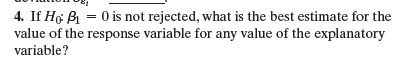×
Get Full Access to Statistics: Informed Decisions Using Data - 5 Edition - Chapter 14.1 - Problem 4
Get Full Access to Statistics: Informed Decisions Using Data - 5 Edition - Chapter 14.1 - Problem 4

×

# ?If H0: b1 = 0 is not rejected, what is the best estimate for the value of the response variable for any value of the explanatory variable?ISBN: 9780134133539 240

## Solution for problem 4 Chapter 14.1

Statistics: Informed Decisions Using Data | 5th Edition

• Textbook Solutions
• 2901 Step-by-step solutions solved by professors and subject experts
• Get 24/7 help from StudySoup virtual teaching assistantsStatistics: Informed Decisions Using Data | 5th Edition

4 5 1 374 Reviews
14
4
Problem 4

If H0: b1 = 0 is not rejected, what is the best estimate for the value of the response variable for any value of the explanatory variable?Step-by-Step Solution:

Step 1 of 5) If H0: b1 = 0 is not rejected, what is the best estimate for the value of the response variable for any value of the explanatory variable Computing Binomial Probabilities via Technology. TI-83/84 Plus Computing P1x2 1. Press 2nd VARS to access the probability distribution menu. 2. Highlight binompdf( and hit ENTER. 3. Enter the number of trials n, the probability of success p, and the number of successes x. Highlight Paste and hit ENTER. For example, with n = 15, p = 0.3, and x = 8, you should see the following on the HOME screen binompdf(15,0.3,8) Then hit ENTER. Computing P1X " x2 1. Press 2nd VARS to access the probability distribution menu. 2. Highlight binomcdf( and hit ENTER. 3. Enter the number of trials n, the probability of success p, and the number of successes x. Highlight Paste and hit ENTER. For example, with n = 15, p = 0.3, and x … 8, you should see the following on the HOME screen binomcdf(15,0.3,8) Then hit ENTER.

Step 2 of 2

## Discover and learn what students are asking

Statistics: Informed Decisions Using Data : Testing the Significance of the Least-Squares Regression Model
?Hurricanes. The following data represent the maximum wind speed (in knots) and atmospheric pressure (in millibars) for a random sample of hurricanes t

Unlock Textbook Solution